# 基于时空FOA-Elman的分布参数系统建模——时空建模Time/Space Separation Based FOA-Elman Modeling for Non-Linear Distributed Parameter Processes—Spatial-Temporal Modeling

DOI: 10.12677/CSA.2019.92038, PDF, HTML, XML, 下载: 382  浏览: 614

Abstract: In this paper, because of infinite-dimensional nature and complex nonlinearities of the distributed parameter systems, a new data-driven modeling method has been proposed. The spatiotemporal output of the system is measured at a finite number of spatial locations; at the same time it is assumed that the input of the system is a temporal variable. Firstly, Karhunen-Loève (K-L) decomposition is used for dimension reduction to get PCA eigenvectors, which can be utilized to extract the nonlinear basis functions in dominant space. After using time-space separation to get the temporal coefficients, then combining the temporal coefficients and the excitation input signal as input and output information, the NARX model can be identified by using Elman neural network whose structure parameters have been optimized by FOA. Finally, model has been reconstructed and the predicted output gotten. Simulation result shows that the proposed modeling method can achieve good performance for distributed parameter systems.

1. 引言

Elman神经网络  是一种动态递归神经网络，其结构简单，运算量小，非常适合于实时非线性动态系统辨识，因此本文使用Elman神经网络对NARX模型进行估计。然而常规Elman神经网络学习算法存在极易陷入局部最优，收敛速度慢等特点，因而考虑使用一种全局优化算法对Elman神经网络的权值和阀值进行全局优化。果蝇优化算法(Fruit Fly Optimization Algorithm, FOA)   是一种新的全局优化算法，具有收敛速度快，精度高的特点。因此，本文使用FOA-Elman神经网络  估计NARX模型。

2. 问题描述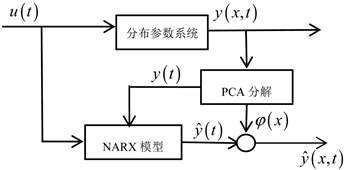Figure 1. Spatial-temporal NARX modelling for distributed parameter system

3. 时空分离及FOA-Elman模型辨识

3.1. 时空分离

3.1.1. 时空分离方法

$y\left(x,t\right)=\underset{i=1}{\overset{\infty }{\sum }}{\phi }_{i}\left(x\right){y}_{i}\left(t\right)$ (1)

$\left({\phi }_{i}\left(x\right),{\phi }_{j}\left(x\right)\right)={\int }_{Ω}{\phi }_{i}\left(x\right){\phi }_{j}\left(x\right)\text{d}x=\left\{\begin{array}{l}0,i\ne j\\ 1,i=j\end{array}$ (2)

$\left\{\begin{array}{l}\underset{\phi i\left(x\right)}{\mathrm{min}}〈\left[y\left(x,t\right)-\underset{i=1}{\overset{n}{\sum }}{\phi }_{i}\left(x\right){\left({\phi }_{i}\left(x\right),y\left(x,t\right)\right)}^{2}\right]〉\\ s.t.\left({\phi }_{i}\left(x\right),{\phi }_{i}\left(x\right)\right)=1,{\phi }_{i}\left(x\right)\in {L}^{2}\left(\Omega \right),i=1,\cdots ,n\end{array}$ (3)

$J=〈\left[y\left(x,t\right)-\underset{i=1}{\overset{n}{\sum }}{\phi }_{i}\left(x\right){\left({\phi }_{i}\left(x\right),y\left(x,t\right)\right)}^{2}\right]〉+\underset{i=1}{\overset{n}{\sum }}{\lambda }_{i}\left(\left({\phi }_{i},{\phi }_{i}\right)-1\right)$ (4)

$\left\{\begin{array}{l}{\int }_{\Omega }R\left(x,\zeta \right){\phi }_{i}\left(\zeta \right)\text{d}\zeta ={\lambda }_{i}{\phi }_{i}\left(x\right),\\ s.t.\left({\phi }_{i},{\phi }_{i}\right)=1,i=1,\cdots ,n\end{array}$ (5)

3.1.2. 快照(Snapshots)法

${\phi }_{i}\left(x\right)=\underset{t=1}{\overset{L}{\sum }}{\gamma }_{it}y\left(x,t\right)$ (6)

${\int }_{\Omega }\frac{1}{L}\underset{t=1}{\overset{L}{\sum }}y\left(x,t\right)y\left(\zeta ,t\right)\underset{k=1}{\overset{L}{\sum }}{\gamma }_{ik}y\left(\zeta ,k\right)\text{d}\zeta ={\lambda }_{i}\underset{t=1}{\overset{L}{\sum }}{\gamma }_{it}y\left(x,t\right)$ (7)

${C}_{tk}=\frac{1}{L}{\int }_{\Omega }y\left(\zeta ,t\right)y\left(\zeta ,k\right)\text{d}\zeta$ (8)

$C{\gamma }_{i}={\lambda }_{i}{\gamma }_{i}$ (9)

${y}_{i}\left(t\right)=\left({\phi }_{i}\left(x\right),y\left(x,t\right)\right),i=1,\cdots ,n$ (10)

${y}_{n}\left(x,t\right)=\underset{i=1}{\overset{n}{\sum }}{\phi }_{i}\left(x\right){y}_{i}\left(t\right)$ (11)

3.1.3. 维数n的选取

${E}_{i}=\frac{{\lambda }_{i}}{\underset{j=1}{\overset{L}{\sum }}{\lambda }_{j}}$ (12)

3.2. FOA-Elman模型辨识

$y\left(t\right)=F\left[y\left(t-1\right),\cdots ,y\left(t-{n}_{y}\right),u\left(t-1\right),\cdots ,u\left(t-{n}_{u}\right)\right]$ (13)

NARX模型为黑箱模型，建模过程无需知道其内部机理，可以利用输入、输出数据建立数学模型。由于神经网络具有强大的非线性逼近能力、快速并行运算能力、较强的容错性和鲁棒性等特点，因而在非线性系统辨识领域有着广泛的应用。其中Elman神经网络是一种典型的动态神经网络，能够直接反映系统的动态特性，广泛应用于动态系统建模中，然而常规Elman神经网络学习算法存在极易陷入局部最优，收敛速度慢等特点，一种新的全局优化算法——FOA算法，可对Elman神经网络的权值和阀值进行全局优化。在本文中，我们将利用基于FOA的Elman神经网络对NARX模型进行辨识。

3.2.1. Elman神经网络

Elman神经网络由四层网络组成：输入层、隐含层(中间层)、承接层和输出层。Elman神经网络是在前馈神经网络的隐含层中增加一个承接层，隐含层的输出通过承接层连接到隐含层的输入。承接层作为一步时移算子使网络具有记忆功能，增强了系统对历史数据的敏感性，进而使模型具有动态处理数据的能力，能直接反映动态过程的系统特性。

Elman神经网络的非线性状态空间表达式如下：

$y\left(k\right)=g\left({w}^{3}x\left(k\right)\right)$ (14)

$x\left(k\right)=f\left({w}^{1}{x}_{c}\left(k\right)\right)+{w}^{2}\left(u\left(k－1\right)\right)$ (15)

${x}_{c}\left(k\right)=x\left(k-1\right)$ (16)

3.2.2. 果蝇优化算法

Step 1：设定群体规模，最大迭代次数，随机初始化果蝇群体位置 $X_axis$$Y_axis$

Step 2：随机果蝇个体搜索方向和搜索距离：

$\left\{\begin{array}{l}{X}_{i}=X_axis+\text{rand}\left(·\right)\\ {Y}_{i}=Y_axis+\text{rand}\left(·\right)\end{array}$ (17)

Step 3：因无法获得食物位置，先计算当前果蝇个体位置与原点的距离，后取距离的倒数作为果蝇个体的味道浓度判定值：

$Dis{t}_{i}=\sqrt{{X}_{i}{}^{2}+{Y}_{i}{}^{2}}$ (18)

 (19)

Step 4：将味道浓度判定值 ${S}_{i}$ 代入适应度函数，求出味道浓度 ${\text{Smell}}_{i}$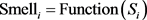(20)

Step 5：找出该果蝇群体中味道浓度值最佳的果蝇个体：

$\left[\text{bestSmell}\text{\hspace{0.17em}}\text{bestindex}\right]=\mathrm{min}\left({\text{Smell}}_{i}\right)$ (21)

Step 6：记录并保留最佳味道浓度值 $\text{bestSmell}$ 与其 $X$$Y$ 坐标，此时果蝇群体将向该位置飞去：

 (22)

Step 7：迭代寻优，重复执行Step 2~Step 6，并判断当前最佳味道浓度值是否优于前一迭代，并且判断是否达到最大迭代次数，若是停止运行。

3.2.3. 基于FOA算法的Elman神经网络预测模型

$\text{RMSE}=\sqrt{\frac{\underset{i=1}{\overset{n}{\sum }}\left({P}_{i}^{r}－{P}_{i}^{f}\right)}{n}}$ (23)

Elman神经网络的相关参数由味道浓度判定值 ${S}_{i}$ 来表示，在每次迭代中，将获得的当前最佳味道浓度判定值 ${S}_{i}$ 代入Elman神经网络进行建模预测，并计算适应度值，重复执行Step 2~Step 6。当FOA算法到达最大迭代次数或终止条件时，迭代终止，得到Elman神经网络最优参数，将网络权值和阀值赋给Elman神经网络，进行训练及预测输出。

$\left\{\begin{array}{l}\stackrel{^}{y}\left(t\right)=\stackrel{^}{F}\left(y\left(t-1\right)\cdots y\left(t-{n}_{y}\right),u\left(t-1\right)\cdots u\left(t-{n}_{u}\right)\right)\\ {\stackrel{^}{y}}_{n}\left(x,t\right)=\underset{i=1}{\overset{n}{\sum }}{\phi }_{i}\left(x\right){\stackrel{^}{y}}_{i}\left(t\right)\end{array}$ (24)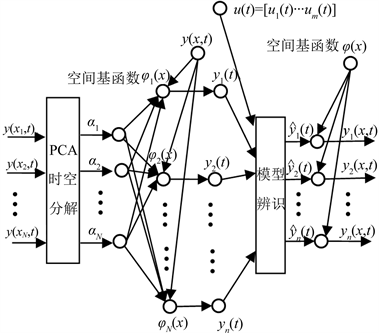Figure 2. Structure chart for spatial-temporal FOA-Elman model

Step 1：为分布参数系统施加激励，获得系统输出 ${\left\{y\left(x,t\right)\right\}}_{i=1,t=1}^{N,L}$ ，利用snapshots法得到系统空间基函数 ${\left\{{\varphi }_{i}\left(x\right)\right\}}_{i=1}^{n}$ 和时间系数 ${\left\{y\left(t\right)\right\}}_{t=1}^{L}$

Step 2：在每个采样点处，对激励信号 $u\left(t\right)$ 和时间系数 $y\left(t\right)$ ，利用FOA-Elman进行模型辨识。

Step 3：系统重构，得到建模输出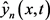4. 仿真研究

4.1. 系统描述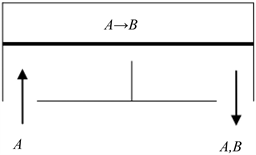Figure 3. Catalytic reaction process

$\frac{\partial y\left(x,t\right)}{\partial t}=\frac{{\partial }^{2}y\left(x,t\right)}{\partial {x}^{2}}+{\beta }_{T}\left({\text{e}}^{-\frac{\gamma }{\left(1+y\right)}}-{e}^{-\gamma }\right)+{\beta }_{u}\left({b}^{T}\left(x\right)u\left(t\right)-y\left(x,t\right)\right)$ (25)

4.2. 时空FOA-Elman建模Table 1. Simulation parameters setting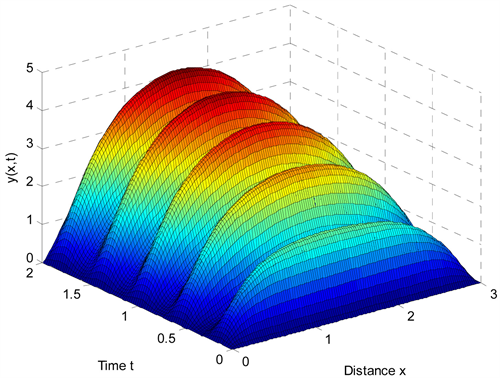Figure 4. Simulation result by finite difference

$\text{RMSE}={\left(\frac{\int \sum e{\left(x,t\right)}^{2}\text{d}x}{\int \text{d}x\sum \Delta t}\right)}^{1/2}$ (26)

FOA-Elman神经网络模型中的模型阶数 ${n}_{y},{n}_{u}$ 分别为3和4。FOA算法参数设置为多次运行最佳值，取Elman神经网络隐含层数量为7。在获得FOA-Elman模型之后，对其使用空间基函数进行时空综合，即可得到完整的时空FOA-Elman神经网络模型。图5$n=4$ 时的空间基函数，表2为取不同个数的空间基函数 $n$ 时FOA-Elman-PCA和Elman-PCA的RMSE对比。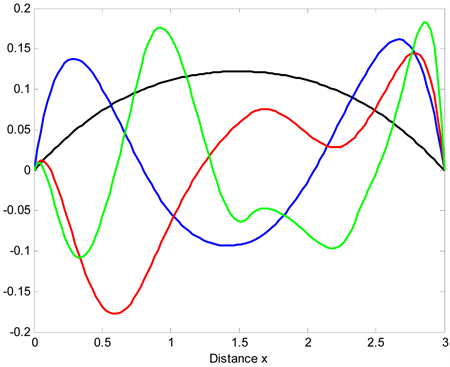Figure 5. Spatial basis function (n = 4)Table 2. RMSE comparison for Elman-PCA and FOA-Elman-PCA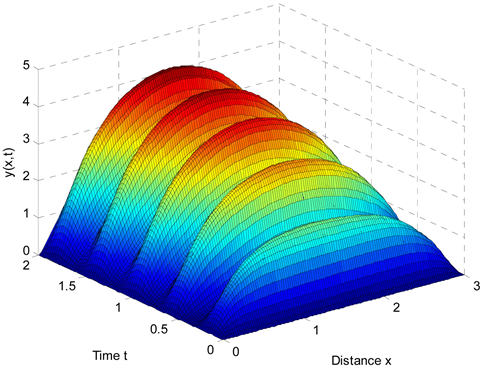Figure 6. Spatial-temporal FOA-Elman model output (n = 3)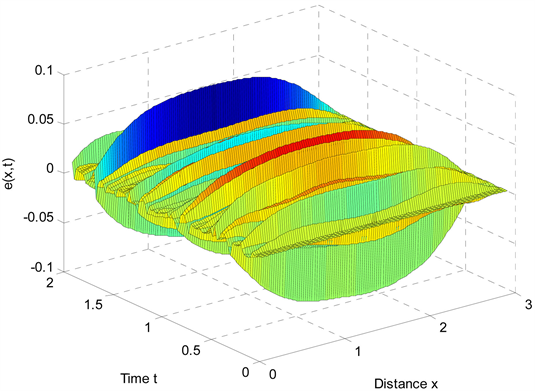Figure 7. Spatial-temporal FOA-Elman model error (n = 3)

5. 结论

  Balas, M.J. (1991) Nonlinear Finite-Dimensional Control of a Class of Nonlinear Distributed Parameter Systems Using Residual-Mode Filters: A Roof of Local Exponential Stability. Journal of Mathematical Analysis & Applications, 162, 63-70. https://doi.org/10.1016/0022-247X(91)90178-3  Balas, M.J. (1983) The Galerkin Method and Feedback Control of Linear Distributed Parameter Systems. Journal of Mathematical Analysis and Applications, 91, 527-546. https://doi.org/10.1016/0022-247X(83)90167-1  Christofides. P.D. and Daoutidis, P. (1997) Finite-Dimensional Control of Parabolic PDE Systems Using Approximate Inertial Manifolds. Journal of Mathematical Analysis & Applications, 216, 398-420. https://doi.org/10.1006/jmaa.1997.5649  Armaou, A. and Christofides, P.D. (2002) Dynamic Optimization of Dissipative PDE Systems Using Nonlinear Order Reduction. Chemical Engineering Science, 57, 50-83. https://doi.org/10.1016/S0009-2509(02)00419-0  Baker, J. and Christofides, P.D. (2000) Finite-Dimensional Approximation and Control of Nonlinear Parabolic PDE Systems. International Journal of Control, 73, 439-456. https://doi.org/10.1080/002071700219614  Li, H.X., Qi, C.K. and Zhang, H.T. (2007) Greatly Enhancing the Modeling Ac-curacy for Distributed Parameter Systems by Nonlinear Time/Space Separation. Physica A, 37, 215-222.  Park, H.M. and Cho, D.H. (1996) The Use of the Karhunen-Loève Decomposition for the Modeling of Distributed Parameter Systems. Chemical Engineer-ing Science, 51, 81-98. https://doi.org/10.1016/0009-2509(95)00230-8  Dai, H., Zheng, Z. and Wang, W. (2017) Nonlinear System Stochastic Response Determination via Fractional Equivalent Linearization and Karhunen-Loève Expansion. Communications in Nonlinear Science & Numerical Simulation, 49, 145-158. https://doi.org/10.1016/j.cnsns.2017.01.033  Mandelj, S., Grabec, I. and Govekar, E. (2001) Statistical Approach to Modeling of Spatiotemporal Dynamics. International Journal of Bifurcation and Chaos, 11, 27-31. https://doi.org/10.1142/S0218127401003802  Coca, D. and Billings, S.A. (2002) Identification of Finite Dimen-sional Models of Infinite Dimensional Dynamical Systems. Automatica, 38, 18-51. https://doi.org/10.1016/S0005-1098(02)00099-7  丛爽, 高雪鹏. 几种递归神经网络及其在系统辨识中应用[J]. 系统工程与电子技术, 2003, 25(2): 194-197.  Pan, W.T. (2012) A New Fruit Fly Optimization Algorithm: Taking the Financial Distress Model as an Example. Knowledge-Based Systems, 26, 69-74. https://doi.org/10.1016/j.knosys.2011.07.001  潘文超. 果蝇最佳化演算法[M]. 台北: 沧海书局, 2011.  韩伟, 王宏华, 等. 基于FOA-Elman神经网络的光伏电站短期出力预测模型[J], 电测与仪表, 2014, 51(12): 120-124.  Sirovich, L. (1991) New Perspectives in Turbulence. Springer, New York. https://doi.org/10.1007/978-1-4612-3156-1  Qi, C.K., Zhang, H.T. and Li, H.X. (2009) A Multi-Channel Spatio-Temporal Hammerstein Modeling Approach for Nonlinear Distributed Parameter Processes. Process Control, 19, 85-99. https://doi.org/10.1016/j.jprocont.2008.01.006  Christofides, P.D. (2001) Nonlinear and Robust Control of PDE Systems: Methods and Applications to Transport-Reaction Processes. Birkhauser, Boston. https://doi.org/10.1007/978-1-4612-0185-4